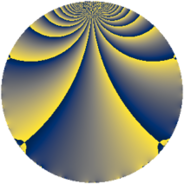Properties

 Label 1368.1.bwLevel $1368$ Weight $1$ Character orbit 1368.bw Rep. character $\chi_{1368}(125,\cdot)$ Character field $\Q(\zeta_{6})$ Dimension $8$ Newform subspaces $1$ Sturm bound $240$ Trace bound $0$

Related objects

Defining parameters

 Level: $$N$$ $$=$$ $$1368 = 2^{3} \cdot 3^{2} \cdot 19$$ Weight: $$k$$ $$=$$ $$1$$ Character orbit: $$[\chi]$$ $$=$$ 1368.bw (of order $$6$$ and degree $$2$$) Character conductor: $$\operatorname{cond}(\chi)$$ $$=$$ $$456$$ Character field: $$\Q(\zeta_{6})$$ Newform subspaces: $$1$$ Sturm bound: $$240$$ Trace bound: $$0$$

Dimensions

The following table gives the dimensions of various subspaces of $$M_{1}(1368, [\chi])$$.

Total New Old
Modular forms 24 8 16
Cusp forms 8 8 0
Eisenstein series 16 0 16

The following table gives the dimensions of subspaces with specified projective image type.

$$D_n$$ $$A_4$$ $$S_4$$ $$A_5$$
Dimension 0 0 8 0

Trace form

 $$8 q - 8 q^{7} + O(q^{10})$$ $$8 q - 8 q^{7} + 4 q^{16} + 4 q^{22} + 4 q^{25} + 8 q^{31} - 4 q^{34} - 8 q^{46} + 4 q^{52} - 8 q^{58} + 4 q^{73} + 4 q^{76} - 4 q^{79} + 8 q^{88} - 8 q^{94} - 8 q^{97} + O(q^{100})$$

Decomposition of $$S_{1}^{\mathrm{new}}(1368, [\chi])$$ into newform subspaces

Label Dim. $$A$$ Field Image CM RM Traces $q$-expansion
$a_{2}$ $a_{3}$ $a_{5}$ $a_{7}$
1368.1.bw.a $8$ $0.683$ $$\Q(\zeta_{24})$$ $S_{4}$ None None $$0$$ $$0$$ $$0$$ $$-8$$ $$q-\zeta_{24}^{5}q^{2}+\zeta_{24}^{10}q^{4}-q^{7}+\zeta_{24}^{3}q^{8}+\cdots$$